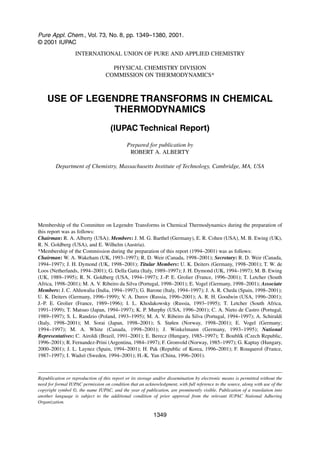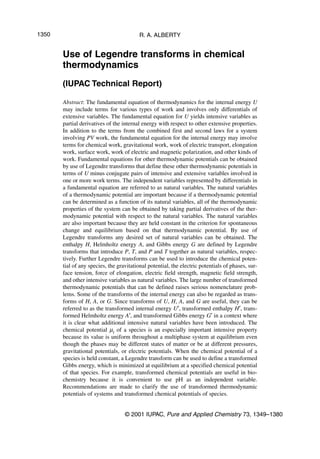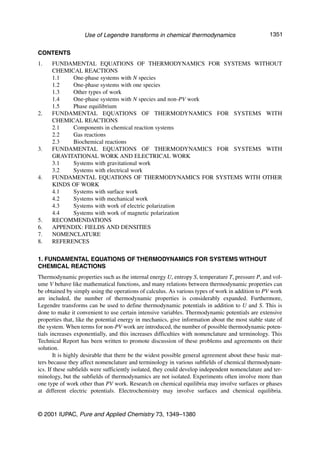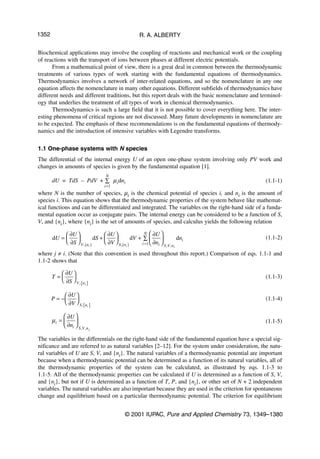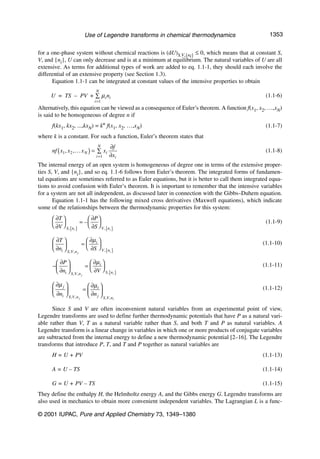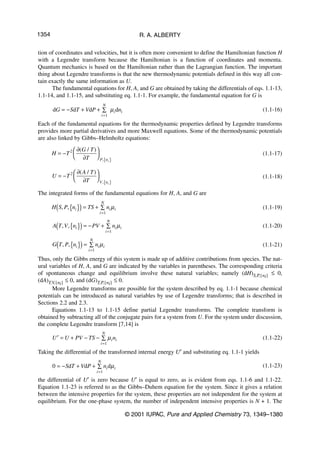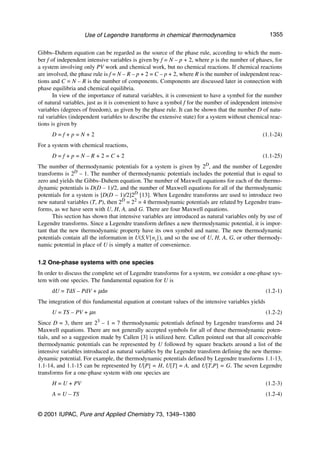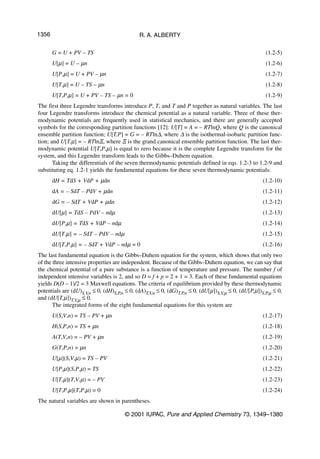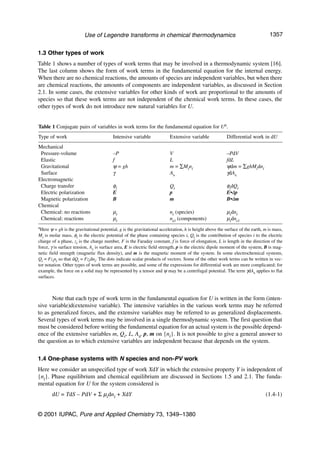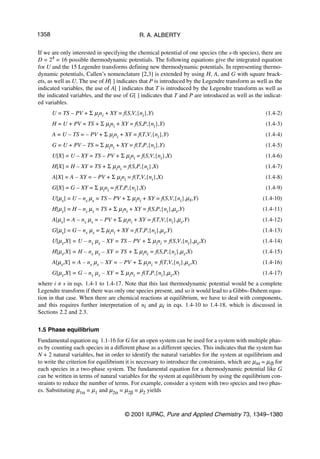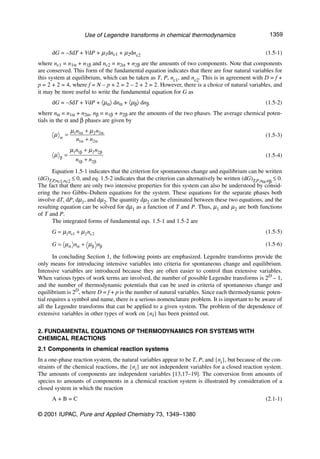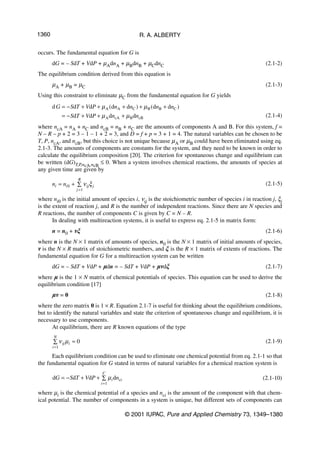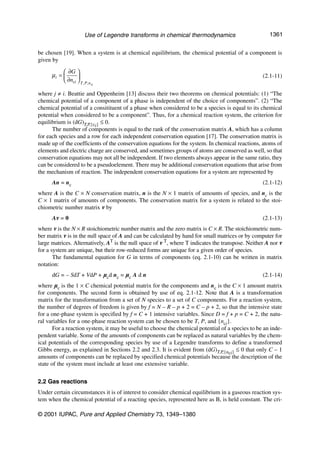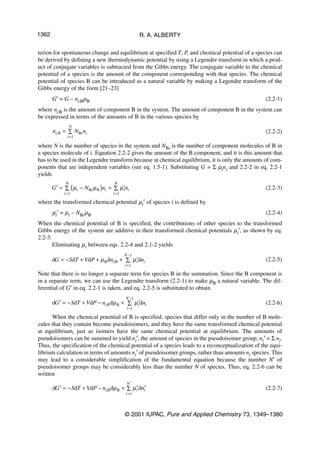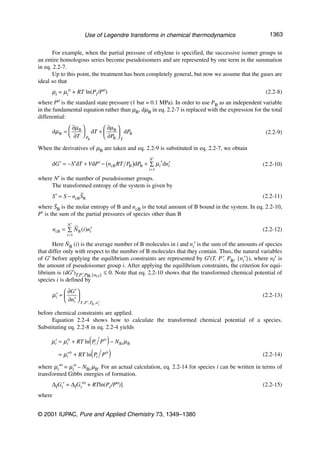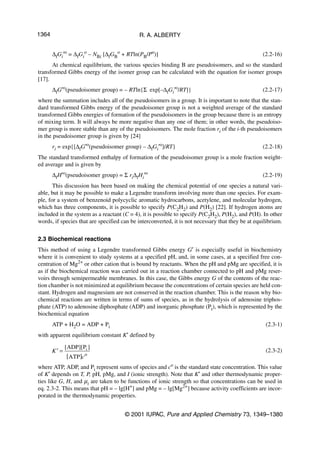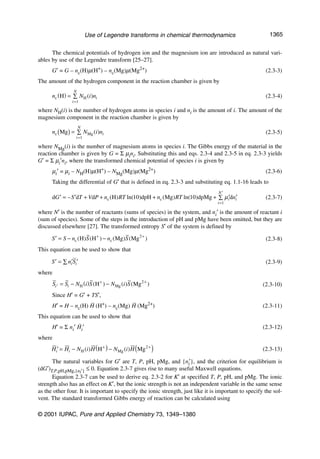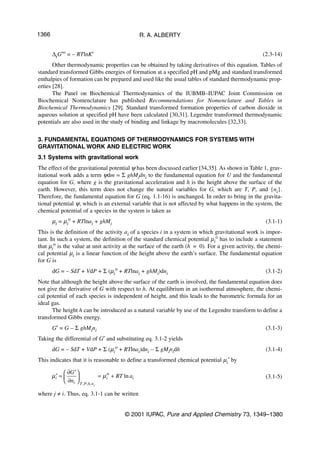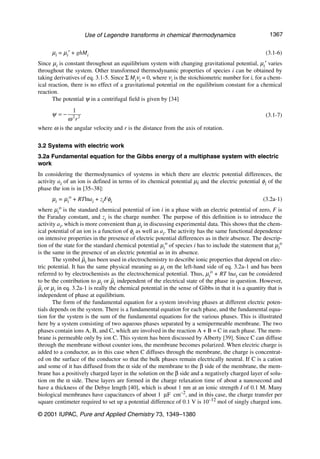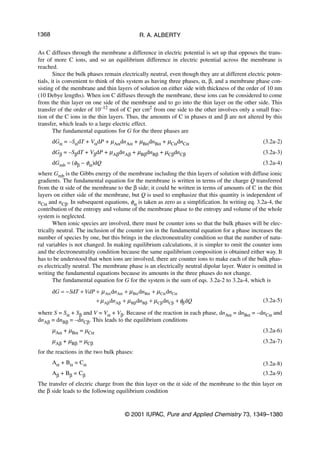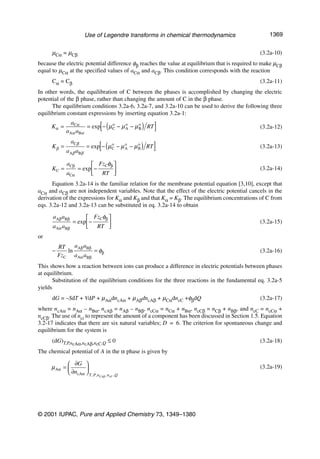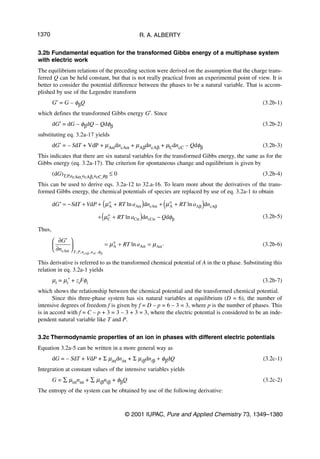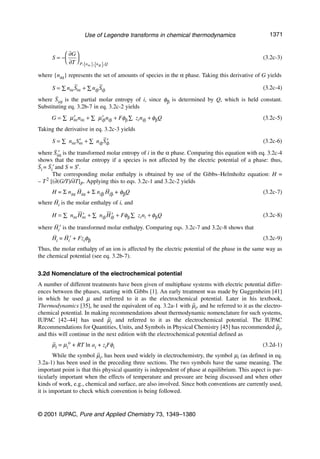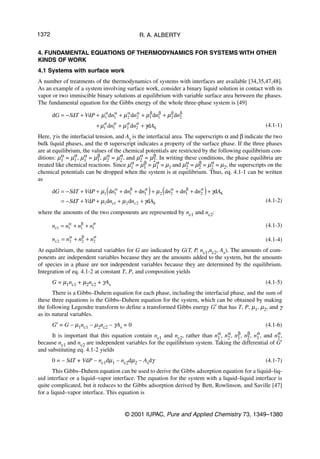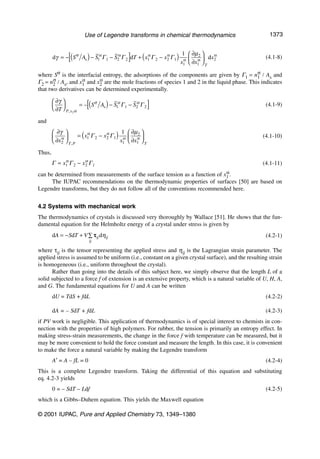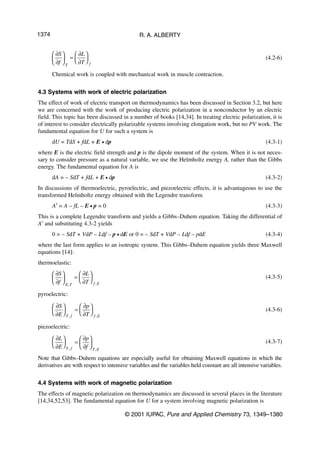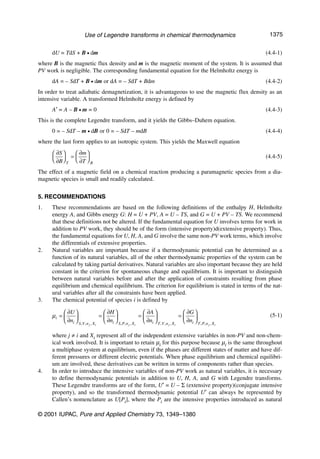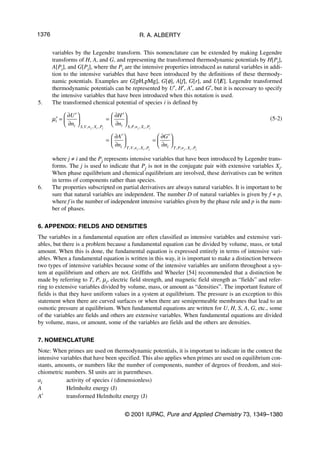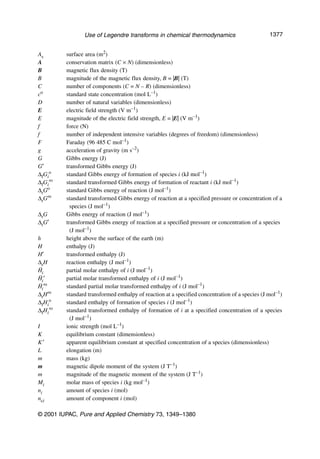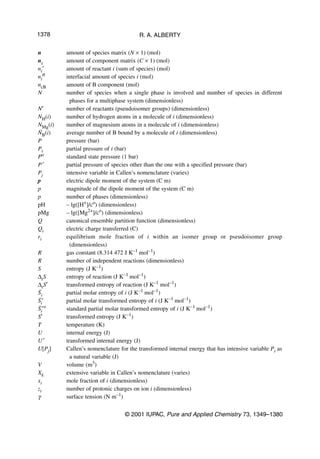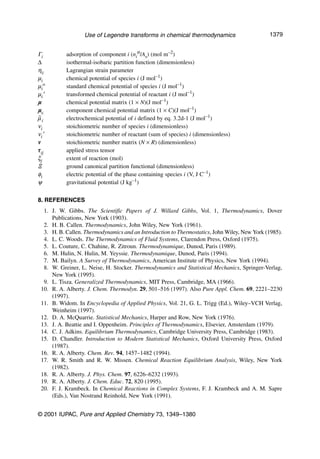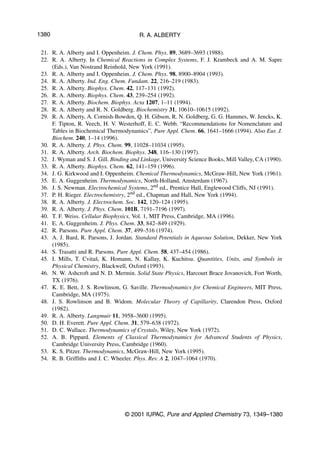Upcoming SlideShare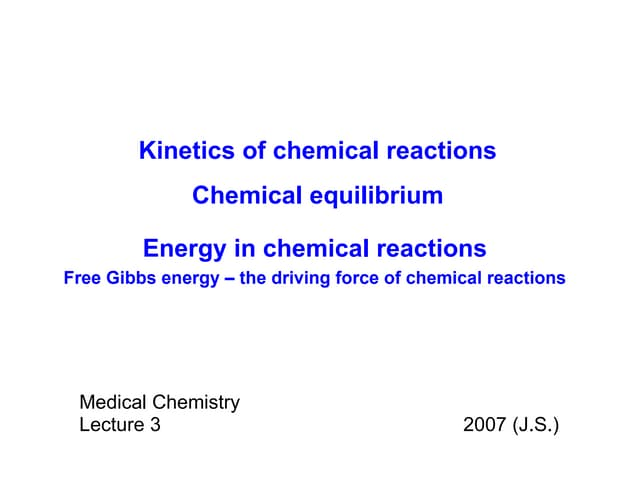03 kinetics _energetics
1 of 32

### 7308x1349

1. Pure Appl. Chem., Vol. 73, No. 8, pp. 1349–1380, 2001. © 2001 IUPAC INTERNATIONAL UNION OF PURE AND APPLIED CHEMISTRY PHYSICAL CHEMISTRY DIVISION COMMISSION ON THERMODYNAMICS* USE OF LEGENDRE TRANSFORMS IN CHEMICAL THERMODYNAMICS (IUPAC Technical Report) Prepared for publication by ROBERT A. ALBERTY Department of Chemistry, Massachusetts Institute of Technology, Cambridge, MA, USA Membership of the Committee on Legendre Transforms in Chemical Thermodynamics during the preparation of this report was as follows: Chairman: R. A. Alberty (USA); Members: J. M. G. Barthel (Germany), E. R. Cohen (USA), M. B. Ewing (UK), R. N. Goldberg (USA), and E. Wilhelm (Austria). *Membership of the Commission during the preparation of this report (1994–2001) was as follows: Chairman: W. A. Wakeham (UK, 1993–1997); R. D. Weir (Canada, 1998–2001); Secretary: R. D. Weir (Canada, 1994–1997); J. H. Dymond (UK, 1998–2001); Titular Members: U. K. Deiters (Germany, 1998–2001); T. W. de Loos (Netherlands, 1994–2001); G. Della Gatta (Italy, 1989–1997); J. H. Dymond (UK, 1994–1997); M. B. Ewing (UK, 1989–1995); R. N. Goldberg (USA, 1994–1997); J.-P. E. Grolier (France, 1996–2001); T. Letcher (South Africa, 1998–2001); M. A. V. Ribeiro da Silva (Portugal, 1998–2001); E. Vogel (Germany, 1998–2001); Associate Members: J. C. Ahluwalia (India, 1994–1997); G. Barone (Italy, 1994–1997); J. A. R. Cheda (Spain, 1998–2001); U. K. Deiters (Germany, 1996–1999); V. A. Durov (Russia, 1996–2001); A. R. H. Goodwin (USA, 1996–2001); J.-P. E. Grolier (France, 1989–1996); I. L. Khodakowsky (Russia, 1993–1995); T. Letcher (South Africa, 1991–1999); T. Matsuo (Japan, 1994–1997); K. P. Murphy (USA, 1996–2001); C. A. Nieto de Castro (Portugal, 1989–1997); S. L. Randzio (Poland, 1993–1995); M. A. V. Ribeiro da Silva (Portugal, 1994–1997); A. Schiraldi (Italy, 1998–2001); M. Sorai (Japan, 1998–2001); S. Stølen (Norway, 1998–2001); E. Vogel (Germany; 1994–1997); M. A. White (Canada, 1998–2001); J. Winkelmann (Germany, 1993–1995); National Representatives: C. Airoldi (Brazil, 1991–2001); E. Berecz (Hungary, 1985–1997); T. Boublík (Czech Republic, 1996–2001); R. Fernandez-Prini (Argentina, 1984–1997); F. Gronvold (Norway, 1985–1997); G. Kaptay (Hungary, 2000–2001); J. L. Laynez (Spain, 1994–2001); H. Pak (Republic of Korea, 1996–2001); F. Rouquerol (France, 1987–1997); I. Wadsö (Sweden, 1994–2001); H.-K. Yan (China, 1996–2001). Republication or reproduction of this report or its storage and/or dissemination by electronic means is permitted without the need for formal IUPAC permission on condition that an acknowledgment, with full reference to the source, along with use of the copyright symbol ©, the name IUPAC, and the year of publication, are prominently visible. Publication of a translation into another language is subject to the additional condition of prior approval from the relevant IUPAC National Adhering Organization. 1349
2. 1350 R. A. ALBERTY Use of Legendre transforms in chemical thermodynamics (IUPAC Technical Report) Abstract: The fundamental equation of thermodynamics for the internal energy U may include terms for various types of work and involves only differentials of extensive variables. The fundamental equation for U yields intensive variables as partial derivatives of the internal energy with respect to other extensive properties. In addition to the terms from the combined first and second laws for a system involving PV work, the fundamental equation for the internal energy may involve terms for chemical work, gravitational work, work of electric transport, elongation work, surface work, work of electric and magnetic polarization, and other kinds of work. Fundamental equations for other thermodynamic potentials can be obtained by use of Legendre transforms that define these other thermodynamic potentials in terms of U minus conjugate pairs of intensive and extensive variables involved in one or more work terms. The independent variables represented by differentials in a fundamental equation are referred to as natural variables. The natural variables of a thermodynamic potential are important because if a thermodynamic potential can be determined as a function of its natural variables, all of the thermodynamic properties of the system can be obtained by taking partial derivatives of the ther- modynamic potential with respect to the natural variables. The natural variables are also important because they are held constant in the criterion for spontaneous change and equilibrium based on that thermodynamic potential. By use of Legendre transforms any desired set of natural variables can be obtained. The enthalpy H, Helmholtz energy A, and Gibbs energy G are defined by Legendre transforms that introduce P, T, and P and T together as natural variables, respec- tively. Further Legendre transforms can be used to introduce the chemical poten- tial of any species, the gravitational potential, the electric potentials of phases, sur- face tension, force of elongation, electric field strength, magnetic field strength, and other intensive variables as natural variables. The large number of transformed thermodynamic potentials that can be defined raises serious nomenclature prob- lems. Some of the transforms of the internal energy can also be regarded as trans- forms of H, A, or G. Since transforms of U, H, A, and G are useful, they can be referred to as the transformed internal energy U′, transformed enthalpy H′, trans- formed Helmholtz energy A′, and transformed Gibbs energy G′ in a context where it is clear what additional intensive natural variables have been introduced. The chemical potential µi of a species is an especially important intensive property because its value is uniform throughout a multiphase system at equilibrium even though the phases may be different states of matter or be at different pressures, gravitational potentials, or electric potentials. When the chemical potential of a species is held constant, a Legendre transform can be used to define a transformed Gibbs energy, which is minimized at equilibrium at a specified chemical potential of that species. For example, transformed chemical potentials are useful in bio- chemistry because it is convenient to use pH as an independent variable. Recommendations are made to clarify the use of transformed thermodynamic potentials of systems and transformed chemical potentials of species. © 2001 IUPAC, Pure and Applied Chemistry 73, 1349–1380
3. Use of Legendre transforms in chemical thermodynamics 1351 CONTENTS 1. FUNDAMENTAL EQUATIONS OF THERMODYNAMICS FOR SYSTEMS WITHOUT CHEMICAL REACTIONS 1.1 One-phase systems with N species 1.2 One-phase systems with one species 1.3 Other types of work 1.4 One-phase systems with N species and non-PV work 1.5 Phase equilibrium 2. FUNDAMENTAL EQUATIONS OF THERMODYNAMICS FOR SYSTEMS WITH CHEMICAL REACTIONS 2.1 Components in chemical reaction systems 2.2 Gas reactions 2.3 Biochemical reactions 3. FUNDAMENTAL EQUATIONS OF THERMODYNAMICS FOR SYSTEMS WITH GRAVITATIONAL WORK AND ELECTRICAL WORK 3.1 Systems with gravitational work 3.2 Systems with electrical work 4. FUNDAMENTAL EQUATIONS OF THERMODYNAMICS FOR SYSTEMS WITH OTHER KINDS OF WORK 4.1 Systems with surface work 4.2 Systems with mechanical work 4.3 Systems with work of electric polarization 4.4 Systems with work of magnetic polarization 5. RECOMMENDATIONS 6. APPENDIX: FIELDS AND DENSITIES 7. NOMENCLATURE 8. REFERENCES 1. FUNDAMENTAL EQUATIONS OF THERMODYNAMICS FOR SYSTEMS WITHOUT CHEMICAL REACTIONS Thermodynamic properties such as the internal energy U, entropy S, temperature T, pressure P, and vol- ume V behave like mathematical functions, and many relations between thermodynamic properties can be obtained by simply using the operations of calculus. As various types of work in addition to PV work are included, the number of thermodynamic properties is considerably expanded. Furthermore, Legendre transforms can be used to define thermodynamic potentials in addition to U and S. This is done to make it convenient to use certain intensive variables. Thermodynamic potentials are extensive properties that, like the potential energy in mechanics, give information about the most stable state of the system. When terms for non-PV work are introduced, the number of possible thermodynamic poten- tials increases exponentially, and this increases difficulties with nomenclature and terminology. This Technical Report has been written to promote discussion of these problems and agreements on their solution. It is highly desirable that there be the widest possible general agreement about these basic mat- ters because they affect nomenclature and terminology in various subfields of chemical thermodynam- ics. If these subfields were sufficiently isolated, they could develop independent nomenclature and ter- minology, but the subfields of thermodynamics are not isolated. Experiments often involve more than one type of work other than PV work. Research on chemical equilibria may involve surfaces or phases at different electric potentials. Electrochemistry may involve surfaces and chemical equilibria. © 2001 IUPAC, Pure and Applied Chemistry 73, 1349–1380
4. 1352 R. A. ALBERTY Biochemical applications may involve the coupling of reactions and mechanical work or the coupling of reactions with the transport of ions between phases at different electric potentials. From a mathematical point of view, there is a great deal in common between the thermodynamic treatments of various types of work starting with the fundamental equations of thermodynamics. Thermodynamics involves a network of inter-related equations, and so the nomenclature in any one equation affects the nomenclature in many other equations. Different subfields of thermodynamics have different needs and different traditions, but this report deals with the basic nomenclature and terminol- ogy that underlies the treatment of all types of work in chemical thermodynamics. Thermodynamics is such a large field that it is not possible to cover everything here. The inter- esting phenomena of critical regions are not discussed. Many future developments in nomenclature are to be expected. The emphasis of these recommendations is on the fundamental equations of thermody- namics and the introduction of intensive variables with Legendre transforms. 1.1 One-phase systems with N species The differential of the internal energy U of an open one-phase system involving only PV work and changes in amounts of species is given by the fundamental equation . N dU = TdS – PdV + ∑ µ i dni (1.1-1) i =1 where N is the number of species, µi is the chemical potential of species i, and ni is the amount of species i. This equation shows that the thermodynamic properties of the system behave like mathemat- ical functions and can be differentiated and integrated. The variables on the right-hand side of a funda- mental equation occur as conjugate pairs. The internal energy can be considered to be a function of S, V, and {ni}, where {ni} is the set of amounts of species, and calculus yields the following relation  ∂U   ∂U  N  ∂U  dU =   dS +   dV + ∑   dni (1.1-2)  ∂S  V ,{n }  ∂V  S,{n } i =1  ∂ni  i i S, V , ni where j ≠ i. (Note that this convention is used throughout this report.) Comparison of eqs. 1.1-1 and 1.1-2 shows that  ∂U  T= (1.1-3)  ∂S  V ,{n } i  ∂U  P=− (1.1-4)  ∂V  S,{n } i  ∂U  µi =   (1.1-5)  ∂ni  S, V , n j The variables in the differentials on the right-hand side of the fundamental equation have a special sig- nificance and are referred to as natural variables [2–12]. For the system under consideration, the natu- ral variables of U are S, V, and {ni}. The natural variables of a thermodynamic potential are important because when a thermodynamic potential can be determined as a function of its natural variables, all of the thermodynamic properties of the system can be calculated, as illustrated by eqs. 1.1-3 to 1.1-5. All of the thermodynamic properties can be calculated if U is determined as a function of S, V, and {ni}, but not if U is determined as a function of T, P, and {ni}, or other set of N + 2 independent variables. The natural variables are also important because they are used in the criterion for spontaneous change and equilibrium based on a particular thermodynamic potential. The criterion for equilibrium © 2001 IUPAC, Pure and Applied Chemistry 73, 1349–1380
5. Use of Legendre transforms in chemical thermodynamics 1353 for a one-phase system without chemical reactions is (dU)S,V,{ni} ≤ 0, which means that at constant S, V, and {ni}, U can only decrease and is at a minimum at equilibrium. The natural variables of U are all extensive. As terms for additional types of work are added to eq. 1.1-1, they should each involve the differential of an extensive property (see Section 1.3). Equation 1.1-1 can be integrated at constant values of the intensive properties to obtain N U = TS – PV + ∑ µ i ni (1.1-6) i =1 Alternatively, this equation can be viewed as a consequence of Euler’s theorem. A function f(x1, x2, …,xN) is said to be homogeneous of degree n if f(kx1, kx2, ...,kxN) = kn f(x1, x2, …,xN) (1.1-7) where k is a constant. For such a function, Euler’s theorem states that N ∂f nf ( x1 , x 2 ,… x N ) = ∑ xi (1.1-8) i =1 ∂xi The internal energy of an open system is homogeneous of degree one in terms of the extensive proper- ties S, V, and {ni}, and so eq. 1.1-6 follows from Euler’s theorem. The integrated forms of fundamen- tal equations are sometimes referred to as Euler equations, but it is better to call them integrated equa- tions to avoid confusion with Euler’s theorem. It is important to remember that the intensive variables for a system are not all independent, as discussed later in connection with the Gibbs–Duhem equation. Equation 1.1-1 has the following mixed cross derivatives (Maxwell equations), which indicate some of the relationships between the thermodynamic properties for this system:  ∂T   ∂P  =– (1.1-9)  ∂V  S,{n }  ∂S  V ,{n } i i  ∂T   ∂µ i    = (1.1-10)  ∂ni  S, V , n  ∂S  V ,{n } j i  ∂P   ∂µ i  −  = (1.1-11)  ∂ni  S, V , n  ∂V  S,{n } j i  ∂µ j   ∂µ    = i (1.1-12)  ∂ni  S, V , n  ∂n j  S, V , n j i Since S and V are often inconvenient natural variables from an experimental point of view, Legendre transforms are used to define further thermodynamic potentials that have P as a natural vari- able rather than V, T as a natural variable rather than S, and both T and P as natural variables. A Legendre transform is a linear change in variables in which one or more products of conjugate variables are subtracted from the internal energy to define a new thermodynamic potential [2–16]. The Legendre transforms that introduce P, T, and T and P together as natural variables are H = U + PV (1.1-13) A = U – TS (1.1-14) G = U + PV – TS (1.1-15) They define the enthalpy H, the Helmholtz energy A, and the Gibbs energy G. Legendre transforms are also used in mechanics to obtain more convenient independent variables. The Lagrangian L is a func- © 2001 IUPAC, Pure and Applied Chemistry 73, 1349–1380
6. 1354 R. A. ALBERTY tion of coordinates and velocities, but it is often more convenient to define the Hamiltonian function H with a Legendre transform because the Hamiltonian is a function of coordinates and momenta. Quantum mechanics is based on the Hamiltonian rather than the Lagrangian function. The important thing about Legendre transforms is that the new thermodynamic potentials defined in this way all con- tain exactly the same information as U. The fundamental equations for H, A, and G are obtained by taking the differentials of eqs. 1.1-13, 1.1-14, and 1.1-15, and substituting eq. 1.1-1. For example, the fundamental equation for G is N dG = − SdT + VdP + ∑ µ i dni (1.1-16) i =1 Each of the fundamental equations for the thermodynamic properties defined by Legendre transforms provides more partial derivatives and more Maxwell equations. Some of the thermodynamic potentials are also linked by Gibbs–Helmholtz equations:  ∂(G / T )  H = −T 2 (1.1-17)  ∂T  P,{n } i  ∂( A / T )  U = −T 2 (1.1-18)  ∂T  V ,{n } i The integrated forms of the fundamental equations for H, A, and G are H ( S, P, {ni }) = TS + ∑ ni µ i N (1.1-19) i =1 A(T , V , {ni }) = − PV + ∑ ni µ i N (1.1-20) i =1 G(T , P, {ni }) = ∑ ni µ i N (1.1-21) i =1 Thus, only the Gibbs energy of this system is made up of additive contributions from species. The nat- ural variables of H, A, and G are indicated by the variables in parentheses. The corresponding criteria of spontaneous change and equilibrium involve these natural variables; namely (dH)S,P,{ni} ≤ 0, (dA)T,V,{ni} ≤ 0, and (dG)T,P,{ni} ≤ 0. More Legendre transforms are possible for the system described by eq. 1.1-1 because chemical potentials can be introduced as natural variables by use of Legendre transforms; that is described in Sections 2.2 and 2.3. Equations 1.1-13 to 1.1-15 define partial Legendre transforms. The complete transform is obtained by subtracting all of the conjugate pairs for a system from U. For the system under discussion, the complete Legendre transform [7,14] is N U ′ = U + PV − TS − ∑ µ i ni (1.1-22) i =1 Taking the differential of the transformed internal energy U′ and substituting eq. 1.1-1 yields N 0 = − SdT + VdP + ∑ ni dµ i (1.1-23) i =1 the differential of U′ is zero because U′ is equal to zero, as is evident from eqs. 1.1-6 and 1.1-22. Equation 1.1-23 is referred to as the Gibbs–Duhem equation for the system. Since it gives a relation between the intensive properties for the system, these properties are not independent for the system at equilibrium. For the one-phase system, the number of independent intensive properties is N + 1. The © 2001 IUPAC, Pure and Applied Chemistry 73, 1349–1380
7. Use of Legendre transforms in chemical thermodynamics 1355 Gibbs–Duhem equation can be regarded as the source of the phase rule, according to which the num- ber f of independent intensive variables is given by f = N – p + 2, where p is the number of phases, for a system involving only PV work and chemical work, but no chemical reactions. If chemical reactions are involved, the phase rule is f = N – R – p + 2 = C – p + 2, where R is the number of independent reac- tions and C = N – R is the number of components. Components are discussed later in connection with phase equilibria and chemical equilibria. In view of the importance of natural variables, it is convenient to have a symbol for the number of natural variables, just as it is convenient to have a symbol f for the number of independent intensive variables (degrees of freedom), as given by the phase rule. It can be shown that the number D of natu- ral variables (independent variables to describe the extensive state) for a system without chemical reac- tions is given by D=f+p=N+2 (1.1-24) For a system with chemical reactions, D=f+p=N–R+2=C+2 (1.1-25) The number of thermodynamic potentials for a system is given by 2D, and the number of Legendre transforms is 2D – 1. The number of thermodynamic potentials includes the potential that is equal to zero and yields the Gibbs–Duhem equation. The number of Maxwell equations for each of the thermo- dynamic potentials is D(D – 1)/2, and the number of Maxwell equations for all of the thermodynamic potentials for a system is [D(D – 1)/2]2D . When Legendre transforms are used to introduce two new natural variables (T, P), then 2D = 22 = 4 thermodynamic potentials are related by Legendre trans- forms, as we have seen with U, H, A, and G. There are four Maxwell equations. This section has shown that intensive variables are introduced as natural variables only by use of Legendre transforms. Since a Legendre transform defines a new thermodynamic potential, it is impor- tant that the new thermodynamic property have its own symbol and name. The new thermodynamic potentials contain all the information in U(S,V{ni}), and so the use of U, H, A, G, or other thermody- namic potential in place of U is simply a matter of convenience. 1.2 One-phase systems with one species In order to discuss the complete set of Legendre transforms for a system, we consider a one-phase sys- tem with one species. The fundamental equation for U is dU = TdS – PdV + µdn (1.2-1) The integration of this fundamental equation at constant values of the intensive variables yields U = TS – PV + µn (1.2-2) Since D = 3, there are 23 – 1 = 7 thermodynamic potentials defined by Legendre transforms and 24 Maxwell equations. There are not generally accepted symbols for all of these thermodynamic poten- tials, and so a suggestion made by Callen  is utilized here. Callen pointed out that all conceivable thermodynamic potentials can be represented by U followed by square brackets around a list of the intensive variables introduced as natural variables by the Legendre transform defining the new thermo- dynamic potential. For example, the thermodynamic potentials defined by Legendre transforms 1.1-13, 1.1-14, and 1.1-15 can be represented by U[P] = H, U[T] = A, and U[T,P] = G. The seven Legendre transforms for a one-phase system with one species are H = U + PV (1.2-3) A = U – TS (1.2-4) © 2001 IUPAC, Pure and Applied Chemistry 73, 1349–1380
8. 1356 R. A. ALBERTY G = U + PV – TS (1.2-5) U[µ] = U – µn (1.2-6) U[P,µ] = U + PV – µn (1.2-7) U[T,µ] = U – TS – µn (1.2-8) U[T,P,µ] = U + PV – TS – µn = 0 (1.2-9) The first three Legendre transforms introduce P, T, and T and P together as natural variables. The last four Legendre transforms introduce the chemical potential as a natural variable. Three of these ther- modynamic potentials are frequently used in statistical mechanics, and there are generally accepted symbols for the corresponding partition functions : U[T] = A = – RTlnQ, where Q is the canonical ensemble partition function; U[T,P] = G = – RTln∆, where ∆ is the isothermal-isobaric partition func- tion; and U[T,µ] = – RTlnΞ, where Ξ is the grand canonical ensemble partition function. The last ther- modynamic potential U[T,P,µ] is equal to zero because it is the complete Legendre transform for the system, and this Legendre transform leads to the Gibbs–Duhem equation. Taking the differentials of the seven thermodynamic potentials defined in eqs. 1.2-3 to 1.2-9 and substituting eq. 1.2-1 yields the fundamental equations for these seven thermodynamic potentials: dH = TdS + VdP + µdn (1.2-10) dA = – SdT – PdV + µdn (1.2-11) dG = – SdT + VdP + µdn (1.2-12) dU[µ] = TdS – PdV – ndµ (1.2-13) dU[P,µ] = TdS + VdP – ndµ (1.2-14) dU[T,µ] = – SdT – PdV – ndµ (1.2-15) dU[T,P,µ] = – SdT + VdP – ndµ = 0 (1.2-16) The last fundamental equation is the Gibbs–Duhem equation for the system, which shows that only two of the three intensive properties are independent. Because of the Gibbs–Duhem equation, we can say that the chemical potential of a pure substance is a function of temperature and pressure. The number f of independent intensive variables is 2, and so D = f + p = 2 + 1 = 3. Each of these fundamental equations yields D(D – 1)/2 = 3 Maxwell equations. The criteria of equilibrium provided by these thermodynamic potentials are (dU)S,V,n ≤ 0, (dH)S,P,n ≤ 0, (dA)T,V,n ≤ 0, (dG)T,P,n ≤ 0, (dU[µ])S,V,µ ≤ 0, (dU[P,µ])S,P,µ ≤ 0, and (dU[T,µ])T,V,µ ≤ 0. The integrated forms of the eight fundamental equations for this system are U(S,V,n) = TS – PV + µn (1.2-17) H(S,P,n) = TS + µn (1.2-18) A(T,V,n) = – PV + µn (1.2-19) G(T,P,n) = µn (1.2-20) U[µ](S,V,µ) = TS – PV (1.2-21) U[P,µ](S,P,µ) = TS (1.2-22) U[T,µ](T,V,µ) = – PV (1.2-23) U[T,P,µ](T,P,µ) = 0 (1.2-24) The natural variables are shown in parentheses. © 2001 IUPAC, Pure and Applied Chemistry 73, 1349–1380
9. Use of Legendre transforms in chemical thermodynamics 1357 1.3 Other types of work Table 1 shows a number of types of work terms that may be involved in a thermodynamic system . The last column shows the form of work terms in the fundamental equation for the internal energy. When there are no chemical reactions, the amounts of species are independent variables, but when there are chemical reactions, the amounts of components are independent variables, as discussed in Section 2.1. In some cases, the extensive variables for other kinds of work are proportional to the amounts of species so that these work terms are not independent of the chemical work terms. In these cases, the other types of work do not introduce new natural variables for U. Table 1 Conjugate pairs of variables in work terms for the fundamental equation for Ua. Type of work Intensive variable Extensive variable Differential work in dU Mechanical Pressure-volume –P V –PdV Elastic f L fdL Gravitational ψ = gh m = ∑Mini ψdm = ∑ghMidni Surface γ As γdAs Electromagnetic Charge transfer φi Qi φidQi Electric polarization E p E•dp Magnetic polarization B m B•dm Chemical Chemical: no reactions µi ni (species) µidni Chemical: reactions µi nci (components) µidnci aHere ψ = gh is the gravitational potential, g is the gravitational acceleration, h is height above the surface of the earth, m is mass, Mi is molar mass, φi is the electric potential of the phase containing species i, Qi is the contribution of species i to the electric charge of a phase, zi is the charge number, F is the Faraday constant, f is force of elongation, L is length in the direction of the force, γ is surface tension, As is surface area, E is electric field strength, p is the electric dipole moment of the system, B is mag- netic field strength (magnetic flux density), and m is the magnetic moment of the system. In some electrochemical systems, Qi = Fzini so that dQi = Fzidni. The dots indicate scalar products of vectors. Some of the other work terms can be written in vec- tor notation. Other types of work terms are possible, and some of the expressions for differential work are more complicated; for example, the force on a solid may be represented by a tensor and ψ may be a centrifugal potential. The term γdAs applies to flat surfaces. Note that each type of work term in the fundamental equation for U is written in the form (inten- sive variable)d(extensive variable). The intensive variables in the various work terms may be referred to as generalized forces, and the extensive variables may be referred to as generalized displacements. Several types of work terms may be involved in a single thermodynamic system. The first question that must be considered before writing the fundamental equation for an actual system is the possible depend- ence of the extensive variables m, Qi, L, As, p, m on {ni}. It is not possible to give a general answer to the question as to which extensive variables are independent because that depends on the system. 1.4 One-phase systems with N species and non-PV work Here we consider an unspecified type of work XdY in which the extensive property Y is independent of {ni}. Phase equilibrium and chemical equilibrium are discussed in Sections 1.5 and 2.1. The funda- mental equation for U for the system considered is dU = TdS – PdV + Σ µidni + XdY (1.4-1) © 2001 IUPAC, Pure and Applied Chemistry 73, 1349–1380
10. 1358 R. A. ALBERTY If we are only interested in specifying the chemical potential of one species (the s-th species), there are D = 24 = 16 possible thermodynamic potentials. The following equations give the integrated equation for U and the 15 Legendre transforms defining new thermodynamic potentials. In representing thermo- dynamic potentials, Callen’s nomenclature [2,3] is extended by using H, A, and G with square brack- ets, as well as U. The use of H[ ] indicates that P is introduced by the Legendre transform as well as the indicated variables, the use of A[ ] indicates that T is introduced by the Legendre transform as well as the indicated variables, and the use of G[ ] indicates that T and P are introduced as well as the indicat- ed variables. U = TS – PV + Σ µini + XY = f(S,V,{ni},Y) (1.4-2) H = U + PV = TS + Σ µini + XY = f(S,P,{ni},Y) (1.4-3) A = U – TS = – PV + Σ µini + XY = f(T,V,{ni},Y) (1.4-4) G = U + PV – TS = Σ µini + XY = f(T,P,{ni},Y) (1.4-5) U[X] = U – XY = TS – PV + Σ µini = f(S,V,{ni},X) (1.4-6) H[X] = H – XY = TS + Σ µini = f(S,P,{ni},X) (1.4-7) A[X] = A – XY = – PV + Σ µini = f(T,V,{ni},X) (1.4-8) G[X] = G – XY = Σ µini = f(T,P,{ni},X) (1.4-9) U[µs] = U – ns µs = TS – PV + Σ µini + XY = f(S,V,{ni},µs,Y) (1.4-10) H[µs] = H – ns µs = TS + Σ µini + XY = f(S,P,{ni},µs,Y) (1.4-11) A[µs] = A – ns µs = – PV + Σ µini + XY = f(T,V,{ni},µs,Y) (1.4-12) G[µs] = G – ns µs = Σ µini + XY = f(T,P,{ni},µs,Y) (1.4-13) U[µs,X] = U – ns µs – XY = TS – PV + Σ µini = f(S,V,{ni},µs,X) (1.4-14) H[µs,X] = H – ns µs – XY = TS + Σ µini = f(S,P,{ni},µs,X) (1.4-15) A[µs,X] = A – ns µs – XY = – PV + Σ µini = f(T,V,{ni},µs,X) (1.4-16) G[µs,X] = G – ns µs – XY = Σ µini = f(T,P,{ni},µs,X) (1.4-17) where i ≠ s in eqs. 1.4-1 to 1.4-17. Note that this last thermodynamic potential would be a complete Legendre transform if there was only one species present, and so it would lead to a Gibbs–Duhem equa- tion in that case. When there are chemical reactions at equilibrium, we have to deal with components, and this requires further interpretation of ni and µi in eqs. 1.4-10 to 1.4-18, which is discussed in Sections 2.2 and 2.3. 1.5 Phase equilibrium Fundamental equation eq. 1.1-16 for G for an open system can be used for a system with multiple phas- es by counting each species in a different phase as a different species. This indicates that the system has N + 2 natural variables, but in order to identify the natural variables for the system at equilibrium and to write the criterion for equilibrium it is necessary to introduce the constraints, which are µiα = µiβ for each species in a two-phase system. The fundamental equation for a thermodynamic potential like G can be written in terms of natural variables for the system at equilibrium by using the equilibrium con- straints to reduce the number of terms. For example, consider a system with two species and two phas- es. Substituting µ1α = µ1 and µ2α = µ2β = µ2 yields © 2001 IUPAC, Pure and Applied Chemistry 73, 1349–1380
11. Use of Legendre transforms in chemical thermodynamics 1359 dG = –SdT + VdP + µ1dnc1 + µ2dnc2 (1.5-1) where nc1 = n1α + n1β and nc2 = n2α + n2β are the amounts of two components. Note that components are conserved. This form of the fundamental equation indicates that there are four natural variables for this system at equilibrium, which can be taken as T, P, nc1, and nc2. This is in agreement with D = f + p = 2 + 2 = 4, where f = N – p + 2 = 2 – 2 + 2 = 2. However, there is a choice of natural variables, and it may be more useful to write the fundamental equation for G as dG = –SdT + VdP + 〈µα〉 dnα + 〈µβ〉 dnβ (1.5-2) where nα = n1α + n2α, nβ = n1β + n2β are the amounts of the two phases. The average chemical poten- tials in the α and β phases are given by µ1n1α + µ 2 n2 α µ α = (1.5-3) n1α + n2 α µ1n1β + µ 2 n2β µ β = (1.5-4) n1β + n2β Equation 1.5-1 indicates that the criterion for spontaneous change and equilibrium can be written (dG)T,P,nc1,nc2 ≤ 0, and eq. 1.5-2 indicates that the criterion can alternatively be written (dG)T,P,nα,nβ ≤ 0. The fact that there are only two intensive properties for this system can also be understood by consid- ering the two Gibbs–Duhem equations for the system. These equations for the separate phases both involve dT, dP, dµ1, and dµ2. The quantity dµ2 can be eliminated between these two equations, and the resulting equation can be solved for dµ1 as a function of T and P. Thus, µ1 and µ2 are both functions of T and P. The integrated forms of fundamental eqs. 1.5-1 and 1.5-2 are G = µ1nc1 + µ 2 nc 2 (1.5-5) G = µ α nα + µβ nβ (1.5-6) In concluding Section 1, the following points are emphasized. Legendre transforms provide the only means for introducing intensive variables into criteria for spontaneous change and equilibrium. Intensive variables are introduced because they are often easier to control than extensive variables. When various types of work terms are involved, the number of possible Legendre transforms is 2D – 1, and the number of thermodynamic potentials that can be used in criteria of spontaneous change and equilibrium is 2D, where D = f + p is the number of natural variables. Since each thermodynamic poten- tial requires a symbol and name, there is a serious nomenclature problem. It is important to be aware of all the Legendre transforms that can be applied to a given system. The problem of the dependence of extensive variables in other types of work on {ni} has been pointed out. 2. FUNDAMENTAL EQUATIONS OF THERMODYNAMICS FOR SYSTEMS WITH CHEMICAL REACTIONS 2.1 Components in chemical reaction systems In a one-phase reaction system, the natural variables appear to be T, P, and {ni}, but because of the con- straints of the chemical reactions, the {ni} are not independent variables for a closed reaction system. The amounts of components are independent variables [13,17–19]. The conversion from amounts of species to amounts of components in a chemical reaction system is illustrated by consideration of a closed system in which the reaction A+B=C (2.1-1) © 2001 IUPAC, Pure and Applied Chemistry 73, 1349–1380
12. 1360 R. A. ALBERTY occurs. The fundamental equation for G is dG = – SdT + VdP + µAdnA + µBdnB + µCdnC (2.1-2) The equilibrium condition derived from this equation is µA + µB = µC (2.1-3) Using this constraint to eliminate µC from the fundamental equation for G yields d G = − SdT + VdP + µ A (dnA + dnC ) + µ B (dnB + dnC ) = − SdT + VdP + µ A dncA + µ BdncB (2.1-4) where ncA = nA + nC and ncB = nB + nC are the amounts of components A and B. For this system, f = N – R – p + 2 = 3 – 1 – 1 + 2 = 3, and D = f + p = 3 + 1 = 4. The natural variables can be chosen to be T, P, ncA, and ncB, but this choice is not unique because µA or µB could have been eliminated using eq. 2.1-3. The amounts of components are constants for the system, and they need to be known in order to calculate the equilibrium composition . The criterion for spontaneous change and equilibrium can be written (dG)T,P,ncA,ncB ≤ 0. When a system involves chemical reactions, the amounts of species at any given time are given by R ni = ni 0 + ∑ ν ij ξ j (2.1-5) j =1 where ni0 is the initial amount of species i, νij is the stoichiometric number of species i in reaction j, ξj is the extent of reaction j, and R is the number of independent reactions. Since there are N species and R reactions, the number of components C is given by C = N – R. In dealing with multireaction systems, it is useful to express eq. 2.1-5 in matrix form: n = n0 + mn (2.1-6) where n is the N × 1 matrix of amounts of species, n0 is the N × 1 matrix of initial amounts of species, m is the N × R matrix of stoichiometric numbers, and n is the R × 1 matrix of extents of reactions. The fundamental equation for G for a multireaction system can be written dG = – SdT + VdP + ldn = – SdT + VdP + lmdn (2.1-7) where l is the 1 × N matrix of chemical potentials of species. This equation can be used to derive the equilibrium condition  lm = 0 (2.1-8) where the zero matrix 0 is 1 × R. Equation 2.1-7 is useful for thinking about the equilibrium conditions, but to identify the natural variables and state the criterion of spontaneous change and equilibrium, it is necessary to use components. At equilibrium, there are R known equations of the type N ∑ ν ij µ i = 0 (2.1-9) i =1 Each equilibrium condition can be used to eliminate one chemical potential from eq. 2.1-1 so that the fundamental equation for G stated in terms of natural variables for a chemical reaction system is C dG = − SdT + VdP + ∑ µ i dnci (2.1-10) i =1 where µi is the chemical potential of a species and nci is the amount of the component with that chem- ical potential. The number of components in a system is unique, but different sets of components can © 2001 IUPAC, Pure and Applied Chemistry 73, 1349–1380
13. Use of Legendre transforms in chemical thermodynamics 1361 be chosen . When a system is at chemical equilibrium, the chemical potential of a component is given by  ∂G  µi =   (2.1-11)  ∂nci  T , P, n cj where j ≠ i. Beattie and Oppenheim  discuss their two theorems on chemical potentials: (1) “The chemical potential of a component of a phase is independent of the choice of components”. (2) “The chemical potential of a consitituent of a phase when considered to be a species is equal to its chemical potential when considered to be a component”. Thus, for a chemical reaction system, the criterion for equilibrium is (dG)T,P,{c } ≤ 0. i The number of components is equal to the rank of the conservation matrix A, which has a column for each species and a row for each independent conservation equation . The conservation matrix is made up of the coefficients of the conservation equations for the system. In chemical reactions, atoms of elements and electric charge are conserved, and sometimes groups of atoms are conserved as well, so that conservation equations may not all be independent. If two elements always appear in the same ratio, they can be considered to be a pseudoelement. There may be additional conservation equations that arise from the mechanism of reaction. The independent conservation equations for a system are represented by An = nc (2.1-12) where A is the C × N conservation matrix, n is the N × 1 matrix of amounts of species, and nc is the C × 1 matrix of amounts of components. The conservation matrix for a system is related to the stoi- chiometric number matrix m by Am = 0 (2.1-13) where m is the N × R stoichiometric number matrix and the zero matrix is C × R. The stoichiometric num- ber matrix m is in the null space of A and can be calculated by hand for small matrices or by computer for large matrices. Alternatively, AT is the null space of m T, where T indicates the transpose. Neither A nor m for a system are unique, but their row-reduced forms are unique for a given order of species. The fundamental equation for G in terms of components (eq. 2.1-10) can be written in matrix notation: dG = – SdT + VdP + lcd nc = lc A d n (2.1-14) where lc is the 1 × C chemical potential matrix for the components and nc is the C × 1 amount matrix for components. The second form is obtained by use of eq. 2.1-12. Note that A is a transformation matrix for the transformation from a set of N species to a set of C components. For a reaction system, the number of degrees of freedom is given by f = N – R – p + 2 = C – p + 2, so that the intensive state for a one-phase system is specified by f = C + 1 intensive variables. Since D = f + p = C + 2, the natu- ral variables for a one-phase reaction system can be chosen to be T, P, and {nci}. For a reaction system, it may be useful to choose the chemical potential of a species to be an inde- pendent variable. Some of the amounts of components can be replaced as natural variables by the chem- ical potentials of the corresponding species by use of a Legendre transforms to define a transformed Gibbs energy, as explained in Sections 2.2 and 2.3. It is evident from (dG)T,P,{nci} ≤ 0 that only C – 1 amounts of components can be replaced by specified chemical potentials because the description of the state of the system must include at least one extensive variable. 2.2 Gas reactions Under certain circumstances it is of interest to consider chemical equilibrium in a gaseous reaction sys- tem when the chemical potential of a reacting species, represented here as B, is held constant. The cri- © 2001 IUPAC, Pure and Applied Chemistry 73, 1349–1380
14. 1362 R. A. ALBERTY terion for spontaneous change and equilibrium at specified T, P, and chemical potential of a species can be derived by defining a new thermodynamic potential by using a Legendre transform in which a prod- uct of conjugate variables is subtracted from the Gibbs energy. The conjugate variable to the chemical potential of a species is the amount of the component corresponding with that species. The chemical potential of species B can be introduced as a natural variable by making a Legendre transform of the Gibbs energy of the form [21–23] G′ = G – ncBµB (2.2-1) where ncB is the amount of component B in the system. The amount of component B in the system can be expressed in terms of the amounts of B in the various species by N ncB = ∑ NBi ni (2.2-2) i =1 where N is the number of species in the system and NBi is the number of component molecules of B in a species molecule of i. Equation 2.2-2 gives the amount of the B component, and it is this amount that has to be used in the Legendre transform because at chemical equilibrium, it is only the amounts of com- ponents that are independent variables (see eq. 1.5-1). Substituting G = Σ µini and 2.2-2 in eq. 2.2-1 yields G ′ = ∑ ( µ i − NBi µ B )ni = ∑ µ i′ni N N (2.2-3) i =1 i =1 where the transformed chemical potential µi′ of species i is defined by µi′ = µi – NBi µB (2.2-4) When the chemical potential of B is specified, the contributions of other species to the transformed Gibbs energy of the system are additive in their transformed chemical potentials µi′, as shown by eq. 2.2-3. Eliminating µi between eqs. 2.2-4 and 2.1-2 yields N −1 dG = − SdT + VdP + µ BdncB + ∑ µ i′dni (2.2-5) i =1 Note that there is no longer a separate term for species B in the summation. Since the B component is in a separate term, we can use the Legendre transform (2.2-1) to make µB a natural variable. The dif- ferential of G′ in eq. 2.2-1 is taken, and eq. 2.2-5 is substituted to obtain N −1 dG ′ = − SdT + VdP − ncBdµ B + ∑ µ i′dni (2.2-6) i =1 When the chemical potential of B is specified, species that differ only in the number of B mole- cules that they contain become pseudoisomers, and they have the same transformed chemical potential at equilibrium, just as isomers have the same chemical potential at equilibrium. The amounts of pseudoisomers can be summed to yield ni′, the amount of species in the pseudoisomer group; ni′ = Σ ni. Thus, the specification of the chemical potential of a species leads to a reconceptualization of the equi- librium calculation in terms of amounts ni′ of pseudoisomer groups, rather than amounts ni species. This may lead to a considerable simplification of the fundamental equation because the number N′ of pseudoisomer groups may be considerably less than the number N of species. Thus, eq. 2.2-6 can be written N′ dG ′ = − SdT + VdP − ncBdµ B + ∑ µ i′dni′ (2.2-7) i =1 © 2001 IUPAC, Pure and Applied Chemistry 73, 1349–1380
15. Use of Legendre transforms in chemical thermodynamics 1363 For example, when the partial pressure of ethylene is specified, the successive isomer groups in an entire homologous series become pseudoisomers and are represented by one term in the summation in eq. 2.2-7. Up to this point, the treatment has been completely general, but now we assume that the gases are ideal so that µi = µio + RT ln(Pi /Po) (2.2-8) Po where is the standard state pressure (1 bar = 0.1 MPa). In order to use PB as an independent variable in the fundamental equation rather than µB, dµB in eq. 2.2-7 is replaced with the expression for the total differential:  ∂µ B   ∂µ  dµ B = dT +  B  dPB (2.2-9)  ∂T  P  ∂PB  B T When the derivatives of µB are taken and eq. 2.2-9 is substituted in eq. 2.2-7, we obtain N′ dG ′ = – S ′dT + VdP ′ − (ncB RT PB )dPB + ∑ µ i ′ dni′ (2.2-10) i =1 where N′ is the number of pseudoisomer groups. The transformed entropy of the system is given by S ′ = S − ncB SB (2.2-11) – where SB is the molar entropy of B and ncB is the total amount of B bound in the system. In eq. 2.2-10, P′ is the sum of the partial pressures of species other than B N′ ncB = ∑ NB (i )ni′ (2.2-12) i =1 – Here NB (i) is the average number of B molecules in i and ni′ is the sum of the amounts of species that differ only with respect to the number of B molecules that they contain. Thus, the natural variables of G′ before applying the equilibrium constraints are represented by G′(T, P′, PB, {ni′}), where ni′ is the amount of pseudoisomer group i. After applying the equilibrium constraints, the criterion for equi- librium is (dG′)T,P′,PB,{nci} ≤ 0. Note that eq. 2.2-10 shows that the transformed chemical potential of species i is defined by  ∂G ′  µi′ =   (2.2-13)  ∂ni′  T , P ′, P B ,n′ j before chemical constraints are applied. Equation 2.2-4 shows how to calculate the transformed chemical potential of a species. Substituting eq. 2.2-8 in eq. 2.2-4 yields ( ) µi′ = µio + RT ln Pi P o − NBi µ B = µ ′ o + RT ln( P P o ) i i (2.2-14) where µi′o = µio – NBi µB. For an actual calculation, eq. 2.2-14 for species i can be written in terms of transformed Gibbs energies of formation. ∆fGi′ = ∆fGi′o + RTln(Pi /Po)] (2.2-15) where © 2001 IUPAC, Pure and Applied Chemistry 73, 1349–1380
16. 1364 R. A. ALBERTY ∆fGi′o = ∆fGio – NBi [∆fGBo + RTln(PB/Po)] (2.2-16) At chemical equilibrium, the various species binding B are pseudoisomers, and so the standard transformed Gibbs energy of the isomer group can be calculated with the equation for isomer groups . ∆fG′o(pseudoisomer group) = – RTln{Σ exp[–∆fGi′o/RT]} (2.2-17) where the summation includes all of the pseudoisomers in a group. It is important to note that the stan- dard transformed Gibbs energy of the pseudoisomer group is not a weighted average of the standard transformed Gibbs energies of formation of the pseudoisomers in the group because there is an entropy of mixing term. It will always be more negative than any one of them; in other words, the pseudoiso- mer group is more stable than any of the pseudoisomers. The mole fraction ri of the i-th pseudoisomers in the pseudoisomer group is given by  ri = exp{[∆fG′o(pseudoisomer group) – ∆fGi′o]/RT} (2.2-18) The standard transformed enthalpy of formation of the pseudoisomer group is a mole fraction weight- ed average and is given by ∆fH′o(pseudoisomer group) = Σ ri∆fHi′o (2.2-19) This discussion has been based on making the chemical potential of one species a natural vari- able, but it may be possible to make a Legendre transform involving more than one species. For exam- ple, for a system of benzenoid polycyclic aromatic hydrocarbons, acetylene, and molecular hydrogen, which has three components, it is possible to specify P(C2H2) and P(H2) . If hydrogen atoms are included in the system as a reactant (C = 4), it is possible to specify P(C2H2), P(H2), and P(H). In other words, if species that are specified can be interconverted, it is not necessary that they be at equilibrium. 2.3 Biochemical reactions This method of using a Legendre transformed Gibbs energy G′ is especially useful in biochemistry where it is convenient to study systems at a specified pH, and, in some cases, at a specified free con- centration of Mg2+ or other cation that is bound by reactants. When the pH and pMg are specified, it is as if the biochemical reaction was carried out in a reaction chamber connected to pH and pMg reser- voirs through semipermeable membranes. In this case, the Gibbs energy G of the contents of the reac- tion chamber is not minimized at equilibrium because the concentrations of certain species are held con- stant. Hydrogen and magnesium are not conserved in the reaction chamber. This is the reason why bio- chemical reactions are written in terms of sums of species, as in the hydrolysis of adenosine triphos- phate (ATP) to adenosine diphosphate (ADP) and inorganic phosphate (Pi), which is represented by the biochemical equation ATP + H2O = ADP + Pi (2.3-1) with apparent equilibrium constant K′ defined by [ADP ][ Pi ] K′ = (2.3-2) [ATP]c o where ATP, ADP, and Pi represent sums of species and co is the standard state concentration. This value of K′ depends on T, P, pH, pMg, and I (ionic strength). Note that K′ and other thermodynamic proper- ties like G, H, and µi are taken to be functions of ionic strength so that concentrations can be used in eq. 2.3-2. This means that pH = – lg[H+] and pMg = – lg[Mg2+] because activity coefficients are incor- porated in the thermodynamic properties. © 2001 IUPAC, Pure and Applied Chemistry 73, 1349–1380
17. Use of Legendre transforms in chemical thermodynamics 1365 The chemical potentials of hydrogen ion and the magnesium ion are introduced as natural vari- ables by use of the Legendre transform [25–27]. G′ = G – nc(H)µ(H+) – nc(Mg)µ(Mg2+) (2.3-3) The amount of the hydrogen component in the reaction chamber is given by N nc (H) = ∑ NH (i )ni (2.3-4) i =1 where NH(i) is the number of hydrogen atoms in species i and ni is the amount of i. The amount of the magnesium component in the reaction chamber is given by N nc (Mg) = ∑ NMg (i )ni (2.3-5) i =1 where NMg(i) is the number of magnesium atoms in species i. The Gibbs energy of the material in the reaction chamber is given by G = Σ µini. Substituting this and eqs. 2.3-4 and 2.3-5 in eq. 2.3-3 yields G′ = Σ µi′ni, where the transformed chemical potential of species i is given by µi′ = µi – NH(H)µ(H+) – NMg(Mg)µ(Mg2+) (2.3-6) Taking the differential of G′ that is defined in eq. 2.3-3 and substituting eq. 1.1-16 leads to N′ dG ′ = – S ′dT + VdP + nc (H) RT ln(10)dpH + nc (Mg) RT ln(10)dpMg + ∑ µ i′dni′ (2.3-7) i =1 where N′ is the number of reactants (sums of species) in the system, and ni′ is the amount of reactant i (sum of species). Some of the steps in the introduction of pH and pMg have been omitted, but they are discussed elsewhere . The transformed entropy S′ of the system is defined by S ′ = S − nc (H)S (H + ) − nc (Mg)S (Mg 2 + ) (2.3-8) This equation can be used to show that S ′ = ∑ ni′Si′ (2.3-9) where Si ′ = Si − NH (i )S (H + ) − NMg (i )S (Mg 2 + ) (2.3-10) Since H′ = G′ + TS′, – – H′ = H – nc(H) H (H+) – nc(Mg) H (Mg2+) (2.3-11) This equation can be used to show that – H′ = Σ ni′ Hi′ (2.3-12) where Hi′ = Hi − NH (i ) H (H + ) − NMg (i ) H (Mg 2 + ) (2.3-13) The natural variables for G′ are T, P, pH, pMg, and {ni′}, and the criterion for equilibrium is (dG′)T,P,pH,pMg,{ni′} ≤ 0. Equation 2.3-7 gives rise to many useful Maxwell equations. Equation 2.3-7 can be used to derive eq. 2.3-2 for K′ at specified T, P, pH, and pMg. The ionic strength also has an effect on K′, but the ionic strength is not an independent variable in the same sense as the other four. It is important to specify the ionic strength, just like it is important to specify the sol- vent. The standard transformed Gibbs energy of reaction can be calculated using © 2001 IUPAC, Pure and Applied Chemistry 73, 1349–1380
18. 1366 R. A. ALBERTY ∆rG′o = – RTlnK′ (2.3-14) Other thermodynamic properties can be obtained by taking derivatives of this equation. Tables of standard transformed Gibbs energies of formation at a specified pH and pMg and standard transformed enthalpies of formation can be prepared and used like the usual tables of standard thermodynamic prop- erties . The Panel on Biochemical Thermodynamics of the IUBMB–IUPAC Joint Commission on Biochemical Nomenclature has published Recommendations for Nomenclature and Tables in Biochemical Thermodynamics . Standard transformed formation properties of carbon dioxide in aqueous solution at specified pH have been calculated [30,31]. Legendre transformed thermodynamic potentials are also used in the study of binding and linkage by macromolecules [32,33]. 3. FUNDAMENTAL EQUATIONS OF THERMODYNAMICS FOR SYSTEMS WITH GRAVITATIONAL WORK AND ELECTRIC WORK 3.1 Systems with gravitational work The effect of the gravitational potential ψ has been discussed earlier [34,35]. As shown in Table 1, grav- itational work adds a term ψdm = Σ ghMidni to the fundamental equation for U and the fundamental equation for G, where g is the gravitational acceleration and h is the height above the surface of the earth. However, this term does not change the natural variables for G, which are T, P, and {ni}. Therefore, the fundamental equation for G (eq. 1.1-16) is unchanged. In order to bring in the gravita- tional potential ψ, which is an external variable that is not affected by what happens in the system, the chemical potential of a species in the system is taken as µi = µio + RTlnai + ghMi (3.1-1) This is the definition of the activity ai of a species i in a system in which gravitational work is impor- tant. In such a system, the definition of the standard chemical potential µio has to include a statement that µio is the value at unit activity at the surface of the earth (h = 0). For a given activity, the chemi- cal potential µi is a linear function of the height above the earth’s surface. The fundamental equation for G is dG = – SdT + VdP + Σ (µio + RTlnai + ghMi)dni (3.1-2) Note that although the height above the surface of the earth is involved, the fundamental equation does not give the derivative of G with respect to h. At equilibrium in an isothermal atmosphere, the chemi- cal potential of each species is independent of height, and this leads to the barometric formula for an ideal gas. The height h can be introduced as a natural variable by use of the Legendre transform to define a transformed Gibbs energy. G′ = G – Σ ghMini (3.1-3) Taking the differential of G′ and substituting eq. 3.1-2 yields dG = – SdT + VdP + Σ (µio + RTlnai)dni – Σ gMinidh (3.1-4) This indicates that it is reasonable to define a transformed chemical potential µi′ by  ∂G ′  µi′ =   = µ io + RT ln ai (3.1-5)  ∂ni  T , P, h, n j where j ≠ i. Thus, eq. 3.1-1 can be written © 2001 IUPAC, Pure and Applied Chemistry 73, 1349–1380
19. Use of Legendre transforms in chemical thermodynamics 1367 µi = µi′ + ghMi (3.1-6) Since µi is constant throughout an equilibrium system with changing gravitational potential, µi′ varies throughout the system. Other transformed thermodynamic properties of species i can be obtained by taking derivatives of eq. 3.1-5. Since Σ Miνi = 0, where νi is the stoichiometric number for i, for a chem- ical reaction, there is no effect of a gravitational potential on the equilibrium constant for a chemical reaction. The potential ψ in a centrifugal field is given by  1 ψ =− (3.1-7) ω 2r 2 where ω is the angular velocity and r is the distance from the axis of rotation. 3.2 Systems with electric work 3.2a Fundamental equation for the Gibbs energy of a multiphase system with electric work In considering the thermodynamics of systems in which there are electric potential differences, the activity ai of an ion is defined in terms of its chemical potential µi and the electric potential φi of the phase the ion is in [35–38]: µi = µio + RTlnai + ziFφi (3.2a-1) where µio is the standard chemical potential of ion i in a phase with an electric potential of zero, F is the Faraday constant, and zi is the charge number. The purpose of this definition is to introduce the activity ai, which is more convenient than µi in discussing experimental data. This shows that the chem- ical potential of an ion is a function of φi as well as ai. The activity has the same functional dependence on intensive properties in the presence of electric potential differences as in their absence. The descrip- tion of the state for the standard chemical potential µio of species i has to include the statement that µio is the same in the presence of an electric potential as in its absence. ~ The symbol µi has been used in electrochemistry to describe ionic properties that depend on elec- tric potential. It has the same physical meaning as µi on the left-hand side of eq. 3.2a-1 and has been referred to by electrochemists as the electrochemical potential. Thus, µio + RT lnai can be considered ~ to be the contribution to µi or µi independent of the electrical state of the phase in question. However, ~ µi or µi in eq. 3.2a-1 is really the chemical potential in the sense of Gibbs in that it is a quantity that is independent of phase at equilibrium. The form of the fundamental equation for a system involving phases at different electric poten- tials depends on the system. There is a fundamental equation for each phase, and the fundamental equa- tion for the system is the sum of the fundamental equations for the various phases. This is illustrated here by a system consisting of two aqueous phases separated by a semipermeable membrane. The two phases contain ions A, B, and C, which are involved in the reaction A + B = C in each phase. The mem- brane is permeable only by ion C. This system has been discussed by Alberty . Since C can diffuse through the membrane without counter ions, the membrane becomes polarized. When electric charge is added to a conductor, as in this case when C diffuses through the membrane, the charge is concentrat- ed on the surface of the conductor so that the bulk phases remain electrically neutral. If C is a cation and some of it has diffused from the α side of the membrane to the β side of the membrane, the mem- brane has a positively charged layer in the solution on the β side and a negatively charged layer of solu- tion on the α side. These layers are formed in the charge relaxation time of about a nanosecond and have a thickness of the Debye length , which is about 1 nm at an ionic strength I of 0.1 M. Many biological membranes have capacitances of about 1 µF cm–2, and in this case, the charge transfer per square centimeter required to set up a potential difference of 0.1 V is 10–12 mol of singly charged ions. © 2001 IUPAC, Pure and Applied Chemistry 73, 1349–1380
20. 1368 R. A. ALBERTY As C diffuses through the membrane a difference in electric potential is set up that opposes the trans- fer of more C ions, and so an equilibrium difference in electric potential across the membrane is reached. Since the bulk phases remain electrically neutral, even though they are at different electric poten- tials, it is convenient to think of this system as having three phases, α, β, and a membrane phase con- sisting of the membrane and thin layers of solution on either side with thickness of the order of 10 nm (10 Debye lengths). When ion C diffuses through the membrane, these ions can be considered to come from the thin layer on one side of the membrane and to go into the thin layer on the other side. This transfer of the order of 10–12 mol of C per cm2 from one side to the other involves only a small frac- tion of the C ions in the thin layers. Thus, the amounts of C in phases α and β are not altered by this transfer, which leads to a large electric effect. The fundamental equations for G for the three phases are dGα = –SαdT + VαdP + µAαdnAα + µBαdnBα + µCαdnCα (3.2a-2) dGβ = –SβdT + VβdP + µAβdnAβ + µBβdnBβ + µCβdnCβ (3.2a-3) dGmb = (φβ − φα)dQ (3.2a-4) where Gmb is the Gibbs energy of the membrane including the thin layers of solution with diffuse ionic gradients. The fundamental equation for the membrane is written in terms of the charge Q transferred from the α side of the membrane to the β side; it could be written in terms of amounts of C in the thin layers on either side of the membrane, but Q is used to emphasize that this quantity is independent of nCα and nCβ. In subsequent equations, φα is taken as zero as a simplification. In writing eq. 3.2a-4, the contribution of the entropy and volume of the membrane phase to the entropy and volume of the whole system is neglected. When ionic species are involved, there must be counter ions so that the bulk phases will be elec- trically neutral. The inclusion of the counter ion in the fundamental equation for a phase increases the number of species by one, but this brings in the electroneutrality condition so that the number of natu- ral variables is not changed. In making equilibrium calculations, it is simpler to omit the counter ions and the electroneutrality condition because the same equilibrium composition is obtained either way. It has to be understood that when ions are involved, there are counter ions to make each of the bulk phas- es electrically neutral. The membrane phase is an electrically neutral dipolar layer. Water is omitted in writing the fundamental equations because its amounts in the three phases do not change. The fundamental equation for G for the system is the sum of eqs. 3.2a-2 to 3.2a-4, which is dG = − SdT + VdP + µ Aα dnAα + µ Bα dnBα + µ Cα dnCα + µ Aβ dnAβ + µ Bβ dnBβ + µ Cβ dnCβ + φβ dQ (3.2a-5) where S = Sα + Sβ and V = Vα + Vβ. Because of the reaction in each phase, dnAα = dnBα = –dnCα and dnAβ = dnBβ = –dnCβ. This leads to the equilibrium conditions µAα + µBα = µCα (3.2a-6) µAβ + µBβ = µCβ (3.2a-7) for the reactions in the two bulk phases: Aα + Bα = Cα (3.2a-8) Aβ + Bβ = Cβ (3.2a-9) The transfer of electric charge from the thin layer on the α side of the membrane to the thin layer on the β side leads to the following equilibrium condition © 2001 IUPAC, Pure and Applied Chemistry 73, 1349–1380
21. Use of Legendre transforms in chemical thermodynamics 1369 µCα = µCβ (3.2a-10) because the electric potential difference φβ reaches the value at equilibrium that is required to make µCβ equal to µCα at the specified values of aCα and aCβ. This condition corresponds with the reaction Cα = Cβ (3.2a-11) In other words, the equilibration of C between the phases is accomplished by changing the electric potential of the β phase, rather than changing the amount of C in the β phase. The equilibrium conditions 3.2a-6, 3.2a-7, and 3.2a-10 can be used to derive the following three equilibrium constant expressions by inserting equation 3.2a-1: Kα = aCα aAα aBα o [( = exp − µ C − µ A − µ B RT o o ) ] (3.2a-12) [( ) ] aCβ Kβ = = exp − µ C − µ A − µ B RT o o o (3.2a-13) aAβ aBβ aCβ  FzCφβ  KC = = exp −  (3.2a-14) aCα  RT  Equation 3.2a-14 is the familiar relation for the membrane potential equation [3,10], except that aCα and aCβ are not independent variables. Note that the effect of the electric potential cancels in the derivation of the expressions for Kα and Kβ and that Kα = Kβ. The equilibrium concentrations of C from eqs. 3.2a-12 and 3.2a-13 can be substituted in eq. 3.2a-14 to obtain aAβ aBβ  FzCφβ  = exp −  (3.2a-15) aAα aBβ  RT  or RT aAβ aBβ − ln = φβ (3.2a-16) FzC aAα aBβ This shows how a reaction between ions can produce a difference in electric potentials between phases at equilibrium. Substitution of the equilibrium conditions for the three reactions in the fundamental eq. 3.2a-5 yields dG = –SdT + VdP + µAαdncAα + µAβdncAβ + µCαdncC +φβdQ (3.2a-17) where ncAα = nAα – nBα, ncAβ = nAβ – nBβ, ncCα = nCα + nBα, ncCβ = nCβ + nBβ, and ncC = ncCα + ncCβ. The use of nci to represent the amount of a component has been discussed in Section 1.5. Equation 3.2-17 indicates that there are six natural variables; D = 6. The criterion for spontaneous change and equilibrium for the system is (dG)T,P,ncAα,ncAβ,ncC,Q ≤ 0 (3.2a-18) The chemical potential of A in the α phase is given by  ∂G  µ Aα =   (3.2a-19)  ∂ncAα  T , P, n CAβ , n cC , Q © 2001 IUPAC, Pure and Applied Chemistry 73, 1349–1380
22. 1370 R. A. ALBERTY 3.2b Fundamental equation for the transformed Gibbs energy of a multiphase system with electric work The equilibrium relations of the preceding section were derived on the assumption that the charge trans- ferred Q can be held constant, but that is not really practical from an experimental point of view. It is better to consider the potential difference between the phases to be a natural variable. That is accom- plished by use of the Legendre transform G′ = G – φβQ (3.2b-1) which defines the transformed Gibbs energy G′. Since dG′ = dG – φβdQ – Qdφβ (3.2b-2) substituting eq. 3.2a-17 yields dG′ = – SdT + VdP + µAαdncAα + µAβdncAβ + µCdncC – Qdφβ (3.2b-3) This indicates that there are six natural variables for the transformed Gibbs energy, the same as for the Gibbs energy (eq. 3.2a-17). The criterion for spontaneous change and equilibrium is given by (dG)T,P,ncAα,ncAβ,ncC,φ β ≤ 0 (3.2b-4) This can be used to derive eqs. 3.2a-12 to 32.a-16. To learn more about the derivatives of the trans- formed Gibbs energy, the chemical potentials of species are replaced by use of eq. 3.2a-1 to obtain o ( o ) ( dG ′ = − SdT + VdP + µ A + RT ln aAα dncAα + µ A + RT ln aAβ dncAβ ) + ( µ C + RT ln aCα )dncCα − Qdφβ o (3.2b-5) Thus,  ∂G ′    = µ A + RT ln aAα = µ Aα ′ o (3.2b-6)  ∂ncAα  T , P, n cAβ , n cC , φ β This derivative is referred to as the transformed chemical potential of A in the α phase. Substituting this relation in eq. 3.2a-1 yields µi = µi′ + ziFφi (3.2b-7) which shows the relationship between the chemical potential and the transformed chemical potential. Since this three-phase system has six natural variables at equilibrium (D = 6), the number of intensive degrees of freedom f is given by f = D – p = 6 – 3 = 3, where p is the number of phases. This is in accord with f = C – p + 3 = 3 – 3 + 3 = 3, where the electric potential is considered to be an inde- pendent natural variable like T and P. 3.2c Thermodynamic properties of an ion in phases with different electric potentials Equation 3.2a-5 can be written in a more general way as dG = – SdT + VdP + Σ µiαdniα + Σ µiβdniβ + φβdQ (3.2c-1) Integration at constant values of the intensive variables yields G = ∑ µiαniα + ∑ µiβniβ + φβQ (3.2c-2) The entropy of the system can be obtained by use of the following derivative: © 2001 IUPAC, Pure and Applied Chemistry 73, 1349–1380
23. Use of Legendre transforms in chemical thermodynamics 1371  ∂G  S=− (3.2c-3)  ∂T  P,{n }, n , Q iα { iβ } where {niα} represents the set of amounts of species in the α phase. Taking this derivative of G yields S = ∑ niα Siα + ∑ niβ Siβ (3.2c-4) – where Siα is the partial molar entropy of i, since φβ is determined by Q, which is held constant. Substituting eq. 3.2b-7 in eq. 3.2c-2 yields G = ∑ µ i′α niα + ∑ µ i′β niβ + Fφβ ∑ zi niβ + φβ Q (3.2c-5) Taking the derivative in eq. 3.2c-3 yields S = ∑ niα Si′α + ∑ niβ Si′ β (3.2c-6) – where Si′α is the transformed molar entropy of i in the α phase. Comparing this equation with eq. 3.2c-4 shows that the molar entropy if a species is not affected by the electric potential of a phase: thus, – – Si = Si′ and S = S′. The corresponding molar enthalpy is obtained by use of the Gibbs–Helmholtz equation: H = – T 2 [(∂(G/T)/∂T]P. Applying this to eqs. 3.2c-1 and 3.2c-2 yields – – H = Σ niα Hiα + Σ niβ Hiβ + φβQ (3.2c-7) – where Hi is the molar enthalpy of i, and H = ∑ niα Hi′ + ∑ niβ Hi′ + Fφβ ∑ zi ni + φβ Q α β (3.2c-8) – where Hi′ is the transformed molar enthalpy. Comparing eqs. 3.2c-7 and 3.2c-8 shows that – – Hi = Hi′ + Fziφβ (3.2c-9) Thus, the molar enthalpy of an ion is affected by the electric potential of the phase in the same way as the chemical potential (see eq. 3.2b-7). 3.2d Nomenclature of the electrochemical potential A number of different treatments have been given of multiphase systems with electric potential differ- ences between the phases, starting with Gibbs . An early treatment was made by Guggenheim  in which he used µ and referred to it as the electrochemical potential. Later in his textbook, ~ Thermodynamics , he used the equivalent of eq. 3.2a-1 with µi, and he referred to it as the electro- chemical potential. In making recommendations about thermodynamic nomenclature for such systems, ~ IUPAC [42–44] has used µi and referred to it as the electrochemical potential. The IUPAC ~ Recommendations for Quantities, Units, and Symbols in Physical Chemistry  has recommended µi, and this will continue in the next edition with the electrochemical potential defined as ~ µi = µio + RT ln ai + ziFφi (3.2d-1) ~ While the symbol µi,has been used widely in electrochemistry, the symbol µi (as defined in eq. 3.2a-1) has been used in the preceding three sections. The two symbols have the same meaning. The important point is that this physical quantity is independent of phase at equilibrium. This aspect is par- ticularly important when the effects of temperature and pressure are being discussed and when other kinds of work, e.g., chemical and surface, are also involved. Since both conventions are currently used, it is important to check which convention is being followed. © 2001 IUPAC, Pure and Applied Chemistry 73, 1349–1380
24. 1372 R. A. ALBERTY 4. FUNDAMENTAL EQUATIONS OF THERMODYNAMICS FOR SYSTEMS WITH OTHER KINDS OF WORK 4.1 Systems with surface work A number of treatments of the thermodynamics of systems with interfaces are available [34,35,47,48]. As an example of a system involving surface work, consider a binary liquid solution in contact with its vapor or two immiscible binary solutions at equilibrium with variable surface area between the phases. The fundamental equation for the Gibbs energy of the whole three-phase system is  α α α α β β β β dG = − SdT + VdP + µ1 dn1 + µ 2 dn2 + µ1 dn1 + µ 2 dn2 σ σ σ σ + µ1 dn1 + µ 2 dn2 + γdAS (4.1-1) Here, γ is the interfacial tension, and As is the interfacial area. The superscripts α and β indicate the two bulk liquid phases, and the σ superscript indicates a property of the surface phase. If the three phases are at equilibrium, the values of the chemical potentials are restricted by the following equilibrium con- α σ α β α σ α β ditions: µ1 = µ1 , µ1 = µ1 , µ2 = µ2 , and µ2 = µ2 . In writing these conditions, the phase equilibria are treated like chemical reactions. Since µ1 α = µ β = µ σ = µ and µ α = µ β = µ σ = µ , the superscripts on the 1 1 1 2 2 2 2 chemical potentials can be dropped when the system is at equilibrium. Thus, eq. 4.1-1 can be written as α ( β σ ) α ( β σ dG = − SdT + VdP + µ1 dn1 + dn1 + dn1 + µ 2 dn2 + dn1 + dn2 + γdAS ) = − SdT + VdP + µ1dnc1 + µ 2 dnc 2 + γdAS (4.1-2) where the amounts of the two components are represented by nc1 and nc2: α β σ nc1 = n1 + n1 + n1 (4.1-3) α β σ nc2 = n2 + n2 + n2 (4.1-4) At equilibrium, the natural variables for G are indicated by G(T, P, nc1,nc2, As). The amounts of com- ponents are independent variables because they are the amounts added to the system, but the amounts of species in a phase are not independent variables because they are determined by the equilibrium. Integration of eq. 4.1-2 at constant T, P, and composition yields G = µ1nc1 + µ2nc2 + γ As (4.1-5) There is a Gibbs–Duhem equation for each phase, including the interfacial phase, and the sum of these three equations is the Gibbs–Duhem equation for the system, which can be obtained by making the following Legendre transform to define a transformed Gibbs energy G′ that has T, P, µ1, µ2, and γ as its natural variables. G′ = G – µ1nc1 – µ2nc2 – γ As = 0 (4.1-6) α α β β σ σ It is important that this equation contain nc1 and nc2, rather than n1 , n2 , n1 , n2 , n1 , and n2 , because nc1 and nc2 are independent variables for the equilibrium system. Taking the differential of G′ and substituting eq. 4.1-2 yields 0 = – SdT + VdP – nc1dµ1 – nc2dµ2 – Asdγ (4.1-7) This Gibbs–Duhem equation can be used to derive the Gibbs adsorption equation for a liquid–liq- uid interface or a liquid–vapor interface. The equation for the system with a liquid–liquid interface is quite complicated, but it reduces to the Gibbs adsorption derived by Bett, Rowlinson, and Saville  for a liquid–vapor interface. This equation is © 2001 IUPAC, Pure and Applied Chemistry 73, 1349–1380
25. Use of Legendre transforms in chemical thermodynamics 1373 [( ) ] dγ = − S σ As − S1α Γ 1 − S2α Γ 2 dT + ( x1 Γ 2 − x 2 Γ 1 ) α α 1 x1α  ∂µ 2   α  dx 2  ∂x1  T α (4.1-8) where Sσ is the interfacial entropy, the adsorptions of the components are given by Γ1 = n1 / As and σ σ α α Γ2 = n2 / As, and x1 and x2 are the mole fractions of species 1 and 2 in the liquid phase. This indicates that two derivatives can be determined experimentally.  ∂γ   ∂T  P, x [( ) = – Sσ As − S1α Γ 1 − S2α Γ 2 ] (4.1-9) 2α and  ∂γ   ∂µ 2  = ( x1 Γ 2 − x 2 Γ 1 ) α α α 1  α  α (4.1-10)  ∂x 2  T , P x1  ∂x1  T Thus, α α Γ = x1 Γ 2 − x 2 Γ 1 (4.1-11) α can be determined from measurements of the surface tension as a function of x1 . The IUPAC recommendations on the thermodynamic properties of surfaces  are based on Legendre transforms, but they do not follow all of the conventions recommended here. 4.2 Systems with mechanical work The thermodynamics of crystals is discussed very thoroughly by Wallace . He shows that the fun- damental equation for the Helmholtz energy of a crystal under stress is given by dA = − SdT + V ∑ τ ij dηij (4.2-1) ij where τij is the tensor representing the applied stress and ηij is the Lagrangian strain parameter. The applied stress is assumed to be uniform (i.e., constant on a given crystal surface), and the resulting strain is homogeneous (i.e., uniform throughout the crystal). Rather than going into the details of this subject here, we simply observe that the length L of a solid subjected to a force f of extension is an extensive property, which is a natural variable of U, H, A, and G. The fundamental equations for U and A can be written dU = TdS + fdL (4.2-2) dA = – SdT + fdL (4.2-3) if PV work is negligible. This application of thermodynamics is of special interest to chemists in con- nection with the properties of high polymers. For rubber, the tension is primarily an entropy effect. In making stress-strain measurements, the change in the force f with temperature can be measured, but it may be more convenient to hold the force constant and measure the length. In this case, it is convenient to make the force a natural variable by making the Legendre transform A′ = A – fL = 0 (4.2-4) This is a complete Legendre transform. Taking the differential of this equation and substituting eq. 4.2-3 yields 0 = – SdT – Ldf (4.2-5) which is a Gibbs–Duhem equation. This yields the Maxwell equation © 2001 IUPAC, Pure and Applied Chemistry 73, 1349–1380